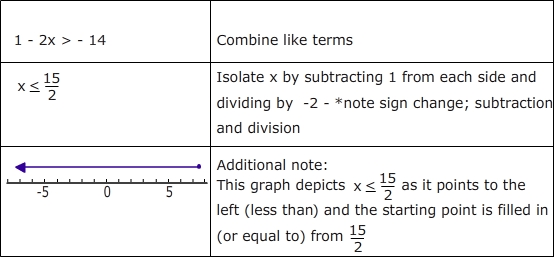# Solving Equations and Inequalities : Solving Equations and Inequalities Quiz

Quiz
*Theme/Title: Solving Equations and Inequalities
* Description/Instructions
To solve equations and inequalities, work to isolate the variable by using inverse operations. Remember, when you multiply or divide an inequality by a negative number, you must reverse the inequality symbol. Solve for the correct value of x in the following equations and inequalities.

For example: -5x + 3x + 1 > -14Group: Algebra Algebra Quizzes
Topic: Solving Equations and Inequalities